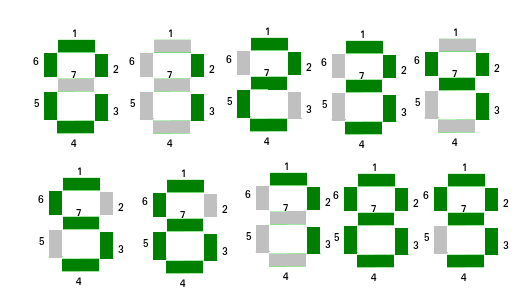# Check if number can be displayed using seven segment led

• Difficulty Level : Medium
• Last Updated : 12 Sep, 2022

Given a string str representing an integer and an integer led which is the count of LEDs available. The task is to check if it is possible to display the number using the given LEDs.
Note that a digit will be displayed as it is displayed on a 7 segment LED. If its possible to display the number then print Yes, otherwise print No.

Here’s an example of seven segment display:Examples:

Input: str = “999”, led = 5
Output: NO
9 takes 6 LEDs to be displayed. So 999 will require 18 LEDs
Since only 5 LEDs are available, it is not possible to display 999

Input: str = “123456789”, led = 43
Output: YES

Input: str = “123456789”, led = 20
Output: NO

Approach: Pre-compute the number of segments used by digits from 0 to 9 and store it. Now for each element of the string count the number of segments used by it. Now, if count ≤ led then print YES else print NO.

The number of segment used by digit:
0 -> 6
1 -> 2
2 -> 5
3 -> 5
4 -> 4
5 -> 5
6 -> 6
7 -> 3
8 -> 7
9 -> 6

Below is the implementation of the above approach:

## C++

 `// C++ implementation of above approach``#include ``using` `namespace` `std;` `// Pre-computed values of segment used by digit 0 to 9.``const` `int` `seg = { 6, 2, 5, 5, 4, 5, 6, 3, 7, 6 };` `// Check if it is possible to display the number``string LedRequired(string s, ``int` `led)``{``    ``int` `count = 0;` `    ``// Finding sum of the segments used by``    ``// each digit of the number``    ``for` `(``int` `i = 0; i < s.length(); ++i) {``        ``count += seg[``int``(s[i]) - 48];``    ``}` `    ``if` `(count <= led)``        ``return` `"YES"``;``    ``else``        ``return` `"NO"``;``}` `// Driven Program``int` `main()``{``    ``string S = ``"123456789"``;``    ``int` `led = 20;` `    ``// Function call to print required answer``    ``cout << LedRequired(S, led) << endl;``    ``return` `0;``}`

## Java

 `// Java implementation of the above approach``public` `class` `GfG{` `    ``// Check if it is possible to display the number``    ``public` `static` `String LedRequired(String s, ``int` `led)``    ``{``        ``// Pre-computed values of segment used by digit 0 to 9.``        ``int` `seg[] = { ``6``, ``2``, ``5``, ``5``, ``4``, ``5``, ``6``, ``3``, ``7``, ``6` `};` `        ``int` `count = ``0``;``        ``// Finding sum of the segments used by``        ``// each digit of the number``        ``for` `(``int` `i = ``0``; i < s.length(); ++i) {``            ``count += seg[(``int``)(s.charAt(i)) - ``48``];``        ``}``      ` `        ``if` `(count <= led)``            ``return` `"YES"``;``        ``else``            ``return` `"NO"``;``    ``}` `    ``public` `static` `void` `main(String []args){``        ` `        ``String S = ``"123456789"``;``        ``int` `led = ``20``;``      ` `        ``// Function call to print required answer``        ``System.out.println(LedRequired(S, led));``    ``}``}` `// This code is contributed by Rituraj Jain`

## Python3

 `# Python3 implementation of above approach` `# Pre-computed values of segment``# used by digit 0 to 9.``seg ``=` `[ ``6``, ``2``, ``5``, ``5``, ``4``,``        ``5``, ``6``, ``3``, ``7``, ``6` `]` `# Check if it is possible to``# display the number``def` `LedRequired(s, led) :` `    ``count ``=` `0` `    ``# Finding sum of the segments used``    ``# by each digit of the number``    ``for` `i ``in` `range``(``len``(s)) :``        ``count ``+``=` `seg[``ord``(s[i]) ``-` `48``]``    ` `    ``if` `(count <``=` `led) :``        ``return` `"YES"``    ``else` `:``        ``return` `"NO"` `# Driver Code``if` `__name__ ``=``=` `"__main__"` `:` `    ``S ``=` `"123456789"``    ``led ``=` `20` `    ``# Function call to print``    ``# required answer``    ``print``(LedRequired(S, led))` `# This code is contributed by Ryuga`

## C#

 `// C# implementation of the above approach``using` `System;``class` `GFG``{` `// Check if it is possible to display the number``public` `static` `String LedRequired(``string` `s, ``int` `led)``{``    ``// Pre-computed values of segment``    ``// used by digit 0 to 9.``    ``int``[] seg = { 6, 2, 5, 5, 4, 5, 6, 3, 7, 6 };` `    ``int` `count = 0;``    ` `    ``// Finding sum of the segments used by``    ``// each digit of the number``    ``for` `(``int` `i = 0; i < s.Length; ++i)``    ``{``        ``count += seg[(``int``)(s[i]) - 48];``    ``}` `    ``if` `(count <= led)``        ``return` `"YES"``;``    ``else``        ``return` `"NO"``;``}` `// Driver Code``public` `static` `void` `Main()``{``    ` `    ``string` `S = ``"123456789"``;``    ``int` `led = 20;` `    ``// Function call to print required answer``    ``Console.WriteLine(LedRequired(S, led));``}``}` `// This code is contributed by Akanksha Rai`

## PHP

 ``

## Javascript

 ``

Output

```NO
```

Complexity Analysis:

• Time Complexity: O(n), where n is the size of the given string
• Auxiliary Space: O(10), as extra space of size 10 is used to create an array

My Personal Notes arrow_drop_up